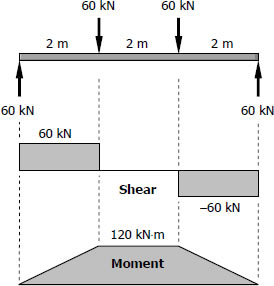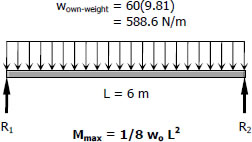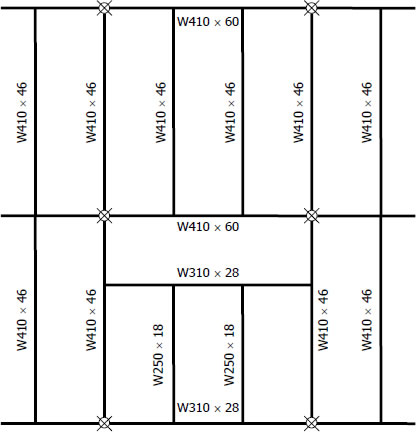# For Girders (G - 2) | Solution to Problem 542

## For Girders (G - 2)$S_{required} = \dfrac{M}{f_b} = \dfrac{120(1000^2)}{120}$
$S_{required} = 1000 \times 10^3 \, \text{mm}^3$

From Appendix B, Table B-2 Properties of Wide-Flange Sections (W Shapes): SI Units, of text book:

 Designation Section Modulus W250 × 89 1100 × 103 mm3 W310 × 74 1060 × 103 mm3 W360 × 64 1030 × 103 mm3 W410 × 60 1060 × 103 mm3 W460 × 60 1120 × 103 mm3

There are two options, both exceeds the required S of 1000 × 103 mm3. One is W410 × 60 with theoretical mass of 59.5 kg/m and the other is W460 × 60 with theoretical mass of 59.6 kg/m. For economic reason,
we prefer W410 × 60.

Checking:$S_{supplied} \ge S_{required} + S_{own-weight}$

$S_{supplied} = 1060 \times 10^3 \, \text{mm}^3$
$S_{required} = 1000 \times 10^3 \, \text{mm}^3$
$S_{own-weight} = \dfrac{M_{own-weight}}{f_b} = \dfrac{\frac{1}{8}(588.6)(6^2)(1000)}{120}$
$S_{own-weight} = 22\,072.5 \, \text{mm}^3$

$S_{required} + S_{own-weight} = (1000 \times 10^3) + 22\,072.5$
$S_{required} + S_{own-weight} = 1\,022\,072.5 \, \text{mm}^3$

$(S_{supplied} = 1060 \times 10^3 \, \text{mm}^3) \gt 1\,022\,072.5 \, \text{mm}^3 \,\,$ (ok!)

Use W410 × 60 for G-2. answer

## Summary:Solution for Beams (B - 1)
Solution for Girder (G - 1)
Solution for Beams (B - 2)
Solution for Beams (B - 3)
Solution for Girders (G - 2)